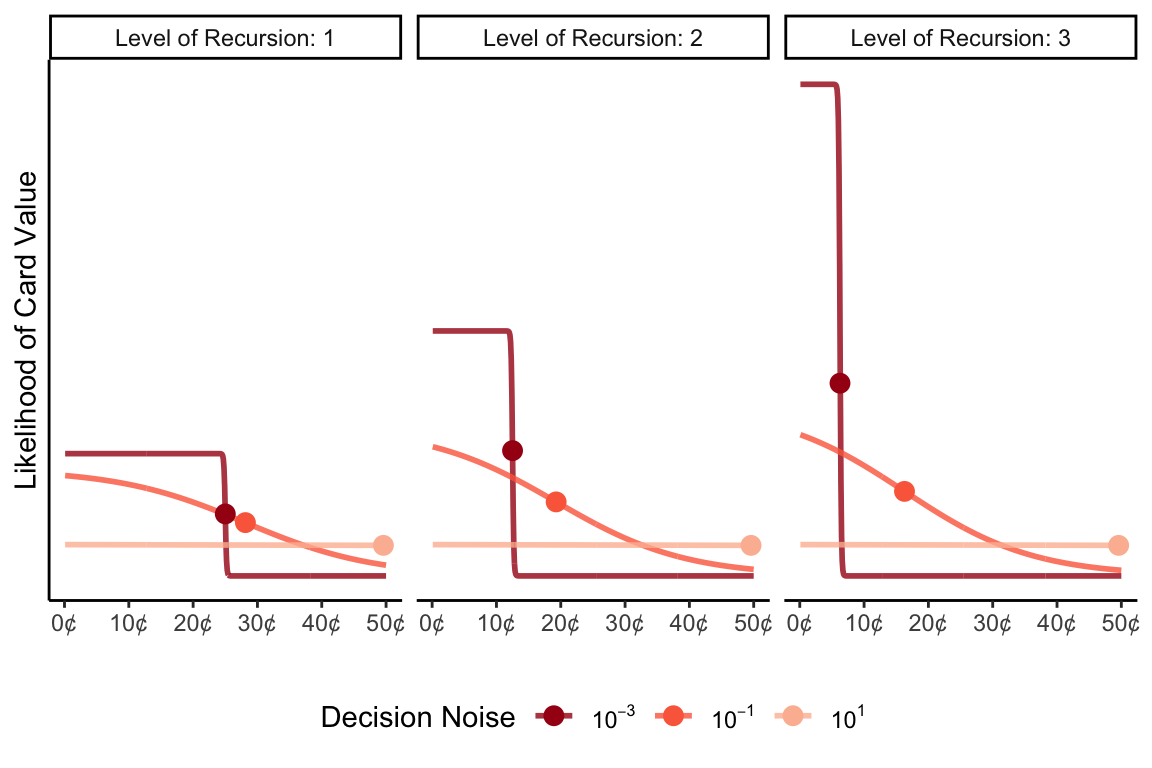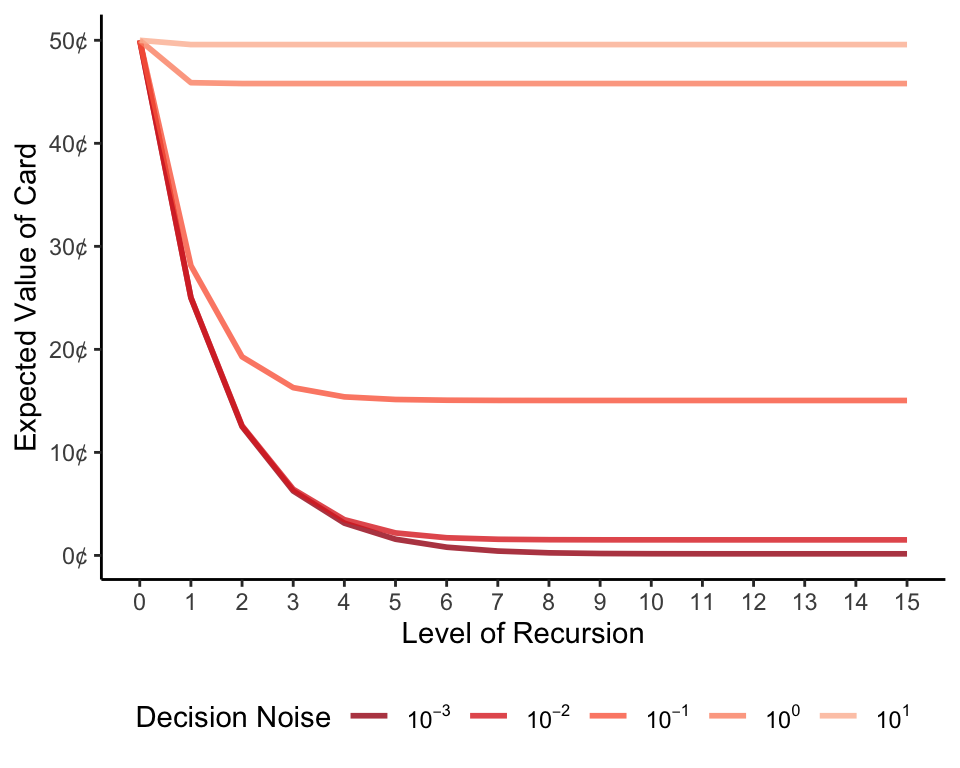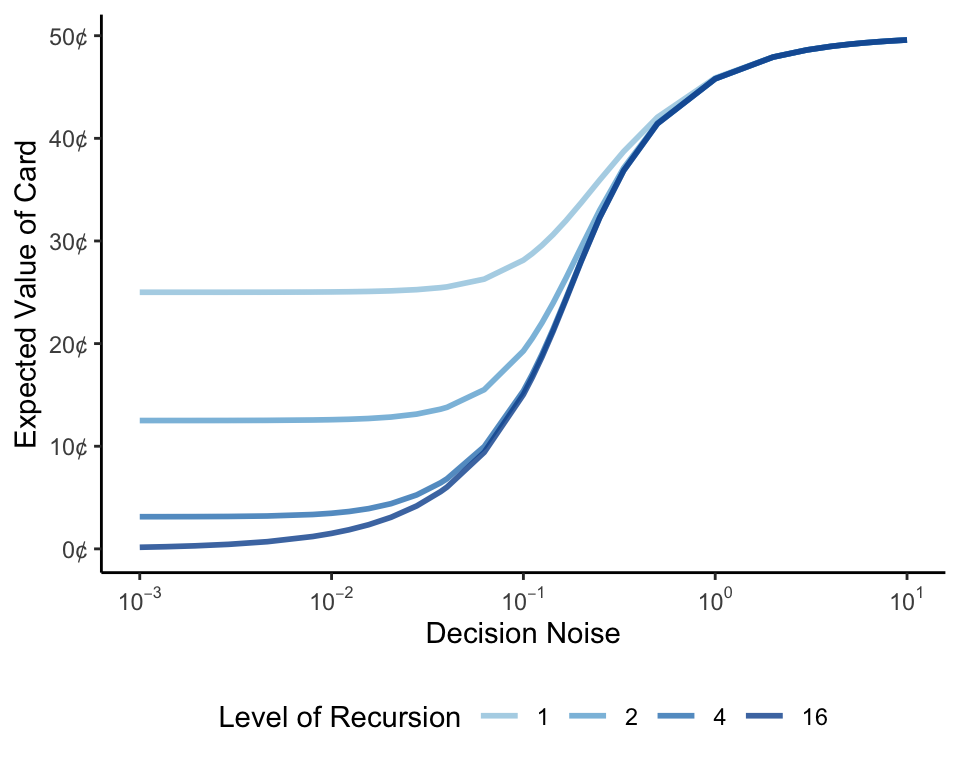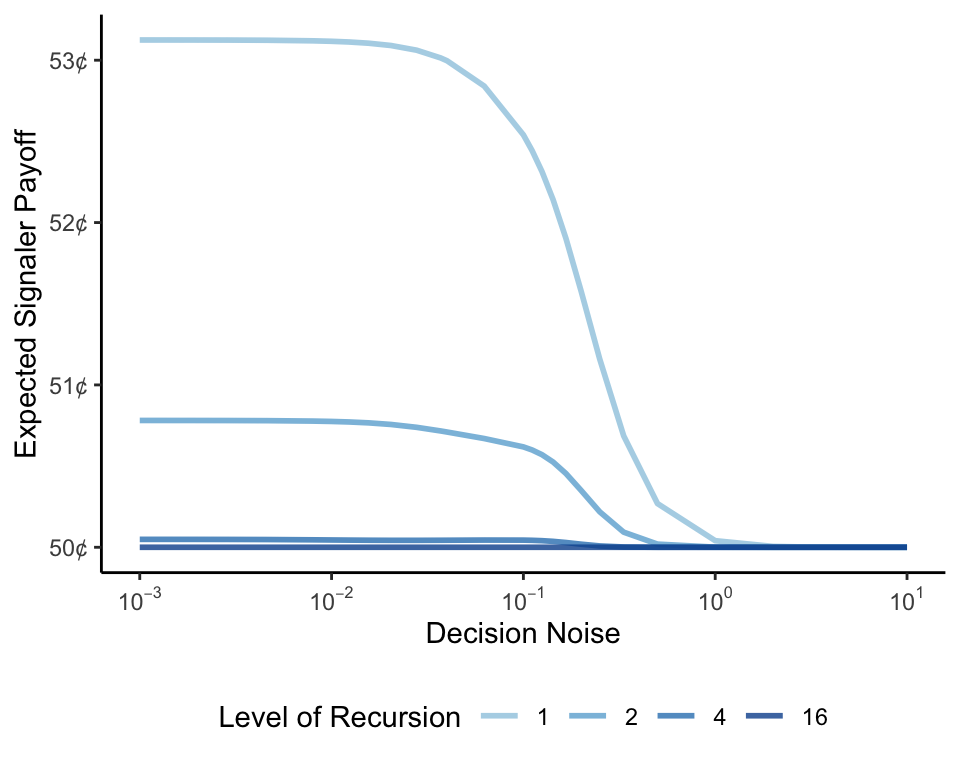# Does failure to reveal information reveal information?

A plea to my future Peloton friends.

Riding my Peloton is one of my favorite hobbies. Sometimes, though, my real-life friends don’t want to be my Peloton friends. They prefer to keep their profiles private because they’re embarrassed by their output and think that I’ll judge them.

But there’s a bigger problem with your potential refusal to friend me. If you reject my request, this decision will reveal new and unflattering information about your skill level.

In other words, if you reject my invitation, you’ll out yourself as the worst Peloton rider imaginable! Isn’t that more embarrassing than just accepting my friend request?

## When unraveling fails

Okay, maybe I don’t actually believe that those who spurn my friend requests are the worst of the worst riders. For one thing, there could be other reasons for you to keep your profile private, such as the desire to hide the fact that you’re spinning during work hours (I do this too). Even if you only worried about my judgment of your fitness, though, the logic of “information unraveling,” which I facetiously outlined above, breaks down under most realistic conditions. Let’s see why.

First, a key assumption of the unraveling logic is that you respond in a deterministically ‘optimal’ way to my inferred beliefs. So, even before I do any fancy recursive theory of mind, if I think that you expect me to estimate your ability as average for your demographic, I assume that you will definitely reveal your quality if you’re above this average and definitely not reveal your quality if you’re below this average. But this assumption is unrealistic for a number of reasons.

For one thing, we each have limited information about what the other believes. That is, in most cases, I can’t perfectly estimate what you think I initially believe your skill level is. Suppose I believe that the typical rider in your demographic outputs an average of 120 watts for a 30-minute ride, and I incorrectly assume that you’d be likely to know that this is my initial guess for your output. Yet, actually, you think that I would estimate your output at 150 watts. If your true output is 130 watts, you might not want to share that information because you believe that you’re below my estimate of 150 watts, even though you’re actually above my estimate of 120 watts.

Even if we do share the same estimate, though, I can’t expect you to be thinking all that carefully about how you compare to my baseline belief about you. While it’s probably the case that — putting humility aside — you’d rather share a surprisingly impressive number than a surprisingly unimpressive one, it’s less obvious how you might respond if your output is close to what we both agree would be my initial guess — especially if you’re a bit shy or overconfident.

There’s a second major issue with the unraveling logic: I must assume that you’re simulating my thought process through many levels of recursion. But this may be cognitively infeasible. Perhaps if you’re just a little below my baseline belief about you, you expect me to lower my expectations about you if you don’t accept my friend request, but you don’t consider the further possibility that I will expect you to make a new decision in light of my lowered expectations. If I can’t assume that you’ll be running through a long chain of recursive inference, then I also can’t assume that you’re at the bottom of the distribution of Peloton riders.

## The hidden number game

We’ve identified two types of explanations for why you might reject my friend request even if you’re not a terrible Peloton rider. First, you might not anticipate or consider that I could simulate several nested levels of theory of mind, which would push my expectations of you towards the bottom. Second, for any given level of recursion, you might not deterministically decide to reveal your quality if and only if you’re better than my prior expectation. Specifically, although I can predict that you would be more eager to friend me if you’re much more skilled than I would expect you to be versus if you’re much less skilled than I would expect you to be, it’s more uncertain what you would decide when your skill level is close to my current estimate.

How much do these two factors — the number of recursive steps and the noisiness of your decision around my expectations — matter? Perhaps even a substantial amount of noise and only a couple recursive steps still leads me to believe you’re a pretty crummy Peloton rider. Or perhaps even a small amount of noise combined with sophisticated theory of mind prevents me from learning about your quality when you reject my request.

To find out, let’s distill my Peloton example into an even simpler game. Here’s how it works. You’re given a card with a uniform random integer between 0 and 100. My task is to guess the number on your card, and I’m rewarded based on how close I get.1 Your goal, however, is to get me to guess the highest number possible, as you will receive a reward equal to my guess, in cents. So if I believe your number is 70, you’ll receive a reward of 70 cents even if your number is actually 30. By analogy, in our simplified world, I want to know your true fitness, while you want me to believe that you’re as fit as possible.

It’s easy to see how we can manipulate depth of theory of mind: we simply carry my chain of reasoning $k$ iterations forward to see how $k$ impacts my final beliefs about the number on your card. For example, you might decide not to reveal your number on the basis of my initial guess that your number is 50 ($k = 1$), but not reconsider whether to reveal now that you believe my guess will be 25 ($k = 2$). If I assume you are only engaging in one level of recursion (and your decision is noiseless), then all I learn is that your number is below 50 — much less than what I would learn if you carried the logic multiple steps further. Keep in mind that, for the sake of these simulations, I’m assuming that we both know how many steps of recursion we each will reason through. While this is unrealistic, it should still give us some sense of how cognitive sophistication impacts the inferences I can draw. Moreover, as I will explain later, it’s sometimes possible through communication to make each step of reasoning common knowledge.

To manipulate your decision noise, we can suppose that, although you’re more likely to show me your number when it is above what you expect me to guess, you sometimes make ‘errors’, especially when your number is close to this expectation. For example, if your number is 55 and you expect me to guess 50, there is some chance (higher than 0%, but less than 50%) that you’ll nevertheless reject my request. Similarly, if your number is 45 and you expect me to guess 50, you might choose to reveal your number anyway (with less than 50% probability). On the other hand, if your number is far from what you expect me to guess (e.g., 90 or 10), you’re more likely to reveal your number if it’s higher than my prediction or reject my request if it’s lower than my prediction.

We can formalize this assumption by assuming that the probability of your decision to show me your number follows a logistic function. Specifically, this function tells us how probable it is that you will reveal your number based on what’s on your card, $x$, and what you believe I would predict if you didn’t reveal it, $x_0$. This function has a noise parameter $\tau$, which encodes the rate at which this probability of revelation transitions from low to high:

$P(\text{reveal})=\frac{1}{1 + e^{-(x-x_0)/\tau}}.$

When $\tau$ is large, your decision is noisy; I can’t assume that you’re definitely going to reveal your number even if it’s quite a bit larger than what you would expect me to guess beforehand. As $\tau$ approaches 0, I expect you to behave more deterministically.2

### Results

Let’s explore the interplay between these two constraints on inference: recursion level and decision noise. All code for the simulations and plots is available here.

Assume that I will use Bayes’ rule to update my beliefs about the number on your card, and you know this when deciding what to do.3 Moreover, because the rules of the game state that your number is a random integer between 0 and 100, we both know that I start with a prior belief that any integer between 0 and 100 is equally likely to be on your card. This allows us to ignore the prior term in Bayes’ rule; my posterior belief that your number is $x$ when you don’t reveal it is simply the probability that you would not reveal your number if it was $x$, normalized to be a probability:

$P(x \mid \text{no reveal},x_0)=\frac{P(\text{no reveal} \mid x,x_0)}{\sum_{x_i=0}^{100}{P(\text{no reveal} \mid x_i,x_0)}},$

where

$\begin{split} P(\text{no reveal} \mid x,x_0) & = 1 - P(\text{reveal} \mid x,x_0) \\ & = 1 - \frac{1}{1 + e^{-(x-x_0)/\tau}}. \end{split}$

Critically, the number that I fill in for $x_0$ will depend on the level of recursion I am simulating for you. Ex ante, my guess for your number is 50, as this is the mean of the uniform distribution from which your number was sampled. So, at the first step of recursion, your decision to (not) reveal is interpreted with respect to this anchor point.

But I may then suppose that you model my first-level inference and calculate my updated expectation of your number once you decide not to reveal. If you did this, your new estimate of my expected guess, $x'_0$, would be the mean of my posterior at the previous step:

$x_0'= \sum_{x_1=0}^{100}x_i*P(x_i \mid \text{no reveal}, x_0=50).$

The more sophisticated version of you would therefore make a decision about whether to reveal your number based on $x_0'$, which leads me to a new posterior belief:

$P(x \mid \text{no reveal},x'_0)=\frac{P(\text{no reveal} \mid x,x'_0)}{\sum_{x_i=0}^{100}{P(\text{no reveal} \mid x_i,x'_0)}}.$

Now I could imagine that you go one step further and use this new posterior to calculate my updated expectation $x''_0$, which leads me to a new posterior, $P(x \mid \text{no reveal}, x''_0)$ — and so on for $k$ steps.

We can implement one step of updating with the following R function. This function takes in an expected guess from the previous step of inference and outputs a new expected guess based on your probability of not revealing your number when using this initial guess. This probability will further depend on the commonly known decision noise parameter, $\tau$, in the logistic function, which can be inputted as the scale in plogis:

recalculate_expectation <- function(x0, tau) {
x <- seq(0, 1, .01) # possible dollar values of your card

# probability you don't reveal your card based on its value
p_no_reveal <- 1 - plogis(x - x0, scale = tau)

# return my new expectation after you reject my request
as.numeric(x %*% (p_no_reveal / sum(p_no_reveal)))
}

To chain this inference in a sequence of recursive steps, we can use the convenient accumulate function from tidyverse to repeatedly apply the recalculate_expectation function, each time using the previous iteration’s output as the input to the next function call:

return_expectations <- function(tau, num_recursions, prior_exp = 0.5) {
guesser_expectations <- accumulate(
seq_len(num_recursions),
$acc, nxt) recalculate_expectation(acc, tau), # nxt is ignored .init = prior_exp ) # return a tibble of the results for all recursive steps tibble( temperature = tau, recursion_level = seq_len(num_recursions + 1) - 1, expectation = guesser_expectations ) } When your decisions are noiseless (i.e., \(\tau=0$), we know that my expectations of your number approach 0 as the number of levels of recursion increase. But it’s less obvious what will happen in the presence of noise. Will my expectation still converge on 0, just at a slower rate? Or will I hit a floor above 0, even in the limit of infinite recursive steps?

Before exploring this question with simulations, a thought experiment might be helpful. Suppose that I knew that you would make the decision about whether to reveal your number completely randomly (e.g., by flipping a coin). In this case, there is no relationship between your number, $x$, and the probability you reveal it. (In the logistic function, this is the limiting case where $\tau$ approaches infinity.) Even if I had the time to run through many recursive levels of theory of mind, this effort would prove futile — I would be stuck guessing that your number is 50. So at least in the special case when your decision is completely random, I hit a floor that is far above 0. This might suggest that at intermediate levels of $\tau$ I also won’t make it all the way to 0.

#### How much do decision noise and recursion matter?

Let’s begin our exploration by focusing on my beliefs after one to three levels of recursion, given that it would be quite cognitively demanding, and therefore surprising, for you or I to recurse much beyond that. Below, I plot my posterior distribution over the number on your card for three different noise levels ($\tau$). The version with $\tau=10^{-3}$ is close to a world in which your decision to reveal is completely deterministic, and the version with $\tau = 10^1$ is close to a world in which your decision to reveal is completely random. The dots on the lines represent my best guess of your number (i.e., the average of my posterior).

As our thought experiment suggested, there seems to be an interaction between decision noise and the level of recursion: when noise is high, recursion barely matters, and when it is low, it matters a lot. Moreover, my expectation of your number barely moves from my prior belief of 50 when your decision noise is high. In contrast, if I know that you are close to deterministic, I am close to guessing 0 even after just three recursive steps. For intermediate decision noise, there are diminishing returns to recursion: the change from level 1 to level 2 matters much more than level 2 to level 3.We can see this pattern of diminishing returns most clearly if we consider my best guess as a function of recursion level. My expectations asymptote earlier, and at a higher guess, for higher noise values. In other words, in the presence of even a moderate amount of decision noise, my cognitive effort is barely rewarded.To make this clearer, we can alternatively plot my best guess as a function of decision noise. When noise is low, the amount of recursion matters; as it increases, however, all of the blue lines converge on my prior belief of 50.In short, to answer our earlier question, it seems that even a small amount of decision noise prevents me from drawing a strong conclusion from your rejection — even if a lot of recursion is possible. Given that most real-world decisions are prone to at least some amount of noise (e.g., because people’s decisions are driven by more than just one factor), this may offer an explanation for why we don’t generally infer much from people’s rejections of requests for information. Moreover, this means that if people do want to hide some unflattering facts about themselves, they can refuse to reveal information without worrying that others will draw strong conclusions from this refusal.

#### Does it pay to be noisy?

We can separately ask a normative question: in this game, is it in your self interest to be a noisy decision maker? This isn’t obvious. If you’re too noisy, then although I will guess that your number is close to 50 when you don’t reveal it, you will also frequently reveal your number when it is below 50 and fail to reveal your number when it is above 50, leading to a lower overall payoff. Perhaps there’s a sweet spot, though, where a little bit of noise leads to the highest expected payoff.

To find out, let’s modify the y-axis from above to plot your expected payoff as a function of decision noise for different recursion levels.4 Recall that you are equally likely to start with any number between 0 and 100, so a world in which you never reveal or always reveal your number would give you an expected payoff of 50 cents. Is there an intermediate level of decision noise that yields a higher expected payoff for you? Surprisingly, the answer seems to be no. Although you can expect to earn more when we go through fewer steps of recursion, you’ll always maximize your expected return by behaving deterministically for any given amount of recursion. In other words, the errors that even a small amount of noise produce will outweigh the benefits of inflating my guess when you don’t show me your number.5In the end, though, regardless of how noisy your decision is, your expected return converges to the baseline of 50 cents for even moderate amounts of recursion. This suggests that your best hope of getting an edge in the game is to credibly signal to me that you can’t, or won’t, think about how your decision influences my beliefs.

#### Playing dumb

It’s frequently remarked that “there are many ways to be wrong, but only one way to be right.” Although this expression is often used to highlight the virtues of rationality, we have seen how it can also harm us in strategic interactions. To be rational is to be predictable in a way that forces us to reveal information that we don’t want to. On the other hand, we have just observed that irrational unpredictability is more harmful than helpful — at least in the context of our simple game.

But could a signaler gain an advantage by only appearing random when it’s in their strategic interest? For example, in the card game, you could maximize your expected payoff by deterministically showing me your number if and only if it is above 50 while pretending that your reason for not showing me your number has nothing to do with its value. In that case, the lowest number I would ever guess is 50, and I would guess a higher number whenever there was such a number on your card that you showed to me.

But just as it’s difficult to fake emotions, it’s difficult to fake ignorance or unpredictability. People learn about randomness by observing erratic behavior; there’s no telltale facial expression or body language that signals this quality — and if there were, it would need to be hard to imitate to remain a reliable signal. Given this, someone who wants to appear random would need to commit to acting in a consistently unpredictable way, which would defeat the purpose.

Could you have more luck signaling that you’re incapable of engaging in recursive theory of mind? We have seen that, if you could signal this, it would give you a small advantage (if your decisions aren’t too noisy). But in some cases, I could prevent this line of reasoning by describing my thought process out loud. You say, “I don’t want to show you my number.” I reply, “OK, then I plan to guess 25 because you’ve signaled that you’re below average. Do you want to show me your number now?” And so on.6

So if you tell me you don’t want to be my Peloton friend, I’ll be sure to point out that this lowers my expectations of you, in hopes that you’ll change your mind.

1. To simplify the math, we can suppose that I want to minimize squared error, and therefore I want to report the expected value (average) of my posterior belief.↩︎

2. I’m assuming here, somewhat unrealistically, that $\tau$ is common knowledge. I don’t expect the results to change a huge amount if it’s not, so long as it’s generally known that you’re relatively more or less random in your decisions.↩︎

3. Of course, the ability to apply Bayes’ rule is itself an assumption of rationality, which could be relaxed, but I will not be exploring this question further.↩︎

4. For this analysis, I assume that you (the signaler) have one more level of theory of mind than I (the guesser) do. That is, you use my $k$-level belief to decide whether to reveal your card, but I don’t further update my guess by modeling your decision at level $k+1$.↩︎

5. Note that this might not be true under different conditions. For example, if it were particularly bad to be outed as having an extremely low number, perhaps decision noise could be more helpful in that type of situation.↩︎

6. You could also imagine playing this game in a big group where everyone had a different number. In that case, the people at the top would reveal their numbers first, prompting the next best to reveal their numbers, and so on. No sophisticated theory of mind is required in this case.↩︎